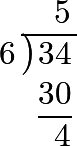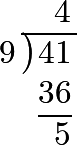### Part I. Section I. Chapter 5. “Of the Division of Simple Quantities.”

45 When a number is to be separated into two, three, or more equal parts, it is done by means of division, which enables us to determine the magnitude of one of those parts. Wlien we wish, for example, to separate the number 12 into three equal parts, we find by division that each of those parts is equal to 4.

The following terms are made use of in this operation. The number which is to be decompounded, or divided, is called the dividend; the number of equal parts sought is called the divisor; the magnitude of one of those parts, determined by the division, is called the quotient: thus, in the above example,

12 is the dividend, or the number to be divided,
3 is the divisor, and
4 is the quotient.

46 It follows from this, that if we divide a number by 2, or into two equal parts, one of those parts, or the quotient, taken twice, makes exactly the number proposed; and, in the same manner, if we have a number to divide by 3, the quotient taken thrice must give the same number again. In general, the multiplication of the quotient by the divisor must always reproduce the dividend.

47 It is for this reason that division is said to be a rule, which teaches us to find a number or quotient, which, being multiplied by the divisor, will exactly produce the dividend. For example, if 35 is to be divided by 5, we seek for a number which, multiplied by 5, will produce 35. Now, this number is 7, since 5 times 7 is 35. The manner of expression employed in this reasoning, is; 5 in 35 goes 7 times; and 5 times 7 makes 35.

48 The dividend therefore may be considered as a product, of which one of the factors is the divisor, and the other the quotient. Thus, supposing we have 63 to divide by 7, we endeavour to find such a product, that, taking 7 for one of its factors, the other factor multiplied by this may exactly give 63. Now 7 · 9 is such a product, and consequently it is the quotient obtained when we divide 63 by 7.

49 In general, if we have to divide a number $$ab$$ by $$a$$, it is evident that the quotient will be $$b$$; for $$a$$ multiplied by $$b$$ gives the dividend $$ab$$. It is clear also, that if we had to divide ab by b, the quotient would be a. And in all examples of division that can be proposed, if we divide the dividend by the quotient, we shall again obtain the divisor; for as 24 divided by 4 gives 6, so 24 divided by 6 will give 4.

50 As the whole operation consists in representing the dividend by two factors, of which one may be equal to the divisor, and the other to the quotient, the following examples will be easily understood. I say first that the dividend $$abc$$, divided by $$a$$, gives $$bc$$; for $$a$$, multiplied by $$bc$$, produces $$abc$$: in the same manner $$abc$$, being divided by $$b$$, we shall have $$ac$$; and $$abc$$, divided by $$ac$$, gives $$b$$. It is also plain, that $$12mn$$, divided by $$3m$$, gives $$4n$$; for $$3m$$, multiplied by $$4n$$, makes $$12mn$$. But if this same number $$12mn$$ had been divided by 12, we should have obtained the quotient $$mn$$.

51 Since every number a may be expressed by $$1a$$, or $$a$$, it is evident that if we had to divide $$a$$, or $$1a$$, by 1, the quotient would be the same number $$a$$. And, on the contrary, if the same number $$a$$, or $$1a$$, is to be divided by $$a$$, the quotient will be 1.

52 It often happens that we cannot represent the dividend as the product of two factors, of which one is equal to the divisor; hence, in this case, the division cannot be performed in the manner we have described.

When we have, for example, 24 to divide by 7, it is at first sight obvious, that the number 7 is not a factor of 24; for the product of 7 · 3 is only 21, and consequently too small; and 7 · 4 makes 28, which is greater than 24. We discover, however, from this, that the quotient must be greater than 3, and less than 4. In order therefore to determine it exactly, we employ another species of numbers, which are called fractions, and which we shall consider in one of the following chapters.

53 Before we proceed to the use of fractions, it is usual to be satisfied with the whole number which approaches nearest to the true quotient, but at the same time paying attention to the remamder which is left; thus we say, 7 in 24 goes 3 times, and the remainder is 3, because 3 times 7 produces only 21, which is 3 less than 24. We may also consider the following examples in the same manner:that is to say, the divisor is 6, the dividend 84, the quotient 5, and the remainder 4.here the divisor is 9, the dividend 41, the quotient 4, and the remainder 5.

The following rule is to be observed in examples where there is a remainder.

54 Multiply the divisor by the quotient, and to the product add the remainder, and the result will be the dividend. This is the method of proving the division, and of discovering whether the calculation is right or not. Thus, in the first of the two last examples, if we multiply 6 by 5, and to the product 30 add the remainder 4, we obtain 34, or the dividend. And in the last example, if we multiply the divisor 9 by the quotient 4, and to the product 36 add the remainder 5, we obtain the dividend 41.

55 Lastly, it is necessary to remark here, with regard to the signs + plus and - minus, that if we divide $$+ab$$ by $$+a$$, the quotient will be $$+b$$, which is evident. But if we divide $$+ab$$ by $$-a$$, the quotient will be $$-b$$; because $$-a$$ multiplied with $$-b$$ gives $$+ab$$. If the dividend is $$-ab$$, and is to be divided by the divisor $$+a$$, the quotient will be $$-b$$; because it is $$-b$$ which, multiplied by $$+a$$, makes $$-ab$$. Lastly, if we have to divide the dividend $$-ab$$ by the divisor $$-a$$, the quotient will be $$+b$$; for the dividend $$-ab$$ is the product of $$-a$$ by $$+b$$.

56 With regard, therefore, to the signs + and -, division requires the same rules to be observed that we have seen take place in multiplication; namely

+ by + makes + ; + by - makes - ;
- by + makes - ; -by - makes + ;

or, in few words, like signs give plus, and unlike signs give minus.

57 Thus, when we divide $$18pq$$ by $$-3p$$, the quotient is $$-6q$$. Farther;

$$-30xy$$ divided by $$+6y$$ gives $$-5x$$, and
$$-54abc$$ divided by $$-9b$$ gives $$+6ac$$;

for, in this last example, $$-9b$$ multiplied by $$+6ac$$ makes $$-6 \cdot 9abc$$, or $$-54abc$$. But enough has been said on the division of simple quantities; we shall therefore hasten to the explanation of fractions, after having added some further remarks on the nature of numbers, with respect to their divisors.

#### Editions

1. Leonhard Euler. Elements of Algebra. Translated by Rev. John Hewlett. Third Edition. Longmans, Hurst, Rees, Orme, and Co. London. 1822.
2. Leonhard Euler. Vollständige Anleitung zur Algebra. Mit den Zusätzen von Joseph Louis Lagrange. Herausgegeben von Heinrich Weber. B. G. Teubner. Leipzig and Berlin. 1911. Leonhardi Euleri Opera omnia. Series prima. Opera mathematica. Volumen primum.